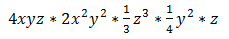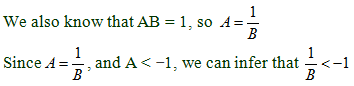# ACT Math Practice Test 3

Difficulty Level – 2: Medium

Directions: Solve each problem and then click on the correct answer. You are permitted to use a calculator on this test.

Congratulations - you have completed . You scored %%SCORE%% out of %%TOTAL%%. Your performance has been rated as %%RATING%%
 Question 1
If x is an even integer and y is an odd integer, which of the following must be an odd integer?

 A 2x + 2y B 2x − 2y C x + y + 1 D x + y + 2 E x − 2y
Question 1 Explanation:
The correct answer is (D). To solve this problem, choose an even integer for x and an odd integer for y and evaluate each of the answer choices. Set x = 0 and y = 1 → only x + y + 2 will evaluate to an odd integer.
 Question 2
35% of 15% of x is equivalent to which of the following?

 A 0.0525x B 0.125x C 0.25x D 0.525x E 5.25x
Question 2 Explanation:
The correct answer is (A). 35% of 15% of a number is 0.35 * 0.15 of that number:
0.35 * 0.15 = 0.0525x
 Question 3
A farmer has a rectangular field that measures 125 feet by 200 feet. He wants to enclose the field with a fence. What is the total length, in feet, he will need for the job?

 A 350 B 450 C 550 D 650 E 750
Question 3 Explanation:
The correct answer is (D). This question is really asking for the perimeter of the field. Add up all of the sides to find the perimeter:
(125 * 2) + (200 * 2) = 650
 Question 4The equation above is equivalent to which of the following?

 A 1⁄3 x y3 z3 B 2⁄3 x3 y5 z5 C 4⁄3 x2 y2 z2 D 3⁄4 x4 y2 z2 E 8⁄3 x4 y2 z3
Question 4 Explanation:
The correct answer is (B). Evaluate the expression to find the most simplified form. First evaluate the coefficients:
4 * 2 * 13 * 14 = 23
x * x2 = x3
y * y2 * y2 = y5
z * z3 * z = z5
Combine each of these terms to get answer choice (B).
 Question 5
What is the slope intercept form of (13x − 5x) + 12 − 2y = 6?

 A y = 3x − 9 B y = 4x + 3 C y = −4x − 3 D y = 6x + 2 E y = −6x + 5
Question 5 Explanation:
The correct answer is (B). Recall that slope-intercept form is y = mx + b where m is the slope and b is the y-intercept. Solve for y:
8x − 2y = −6
2y = 8x + 6
Divide everything by 2:
y = 4x + 3
 Question 6
A and B are reciprocals (when multiplied together their product is 1). If A < −1, then B must be which of the following?

 A B > 1 B B < 0 C 0 < B < 1 D −1 < B < 0 E B < −1
Question 6 Explanation:
The correct answer is (D). If the product of two numbers is positive, the two numbers must have the same sign. That is, if ab > 0, then either a > 0 and b > 0, or a < 0 and b < 0.

We are told that A < −1 (which implies that A < 0).
So we know that B < 0.If we take reciprocals on both sides of the last inequality, we must flip the inequality sign. Hence: B > −1

So we know that B < 0, and B > −1. We can represent this as a compound inequality: −1 < B < 0
 Question 7
Magazine subscriptions cost \$9.99 a month per magazine after an initial contract fee of \$24.99. Which expression represents the cost of m magazines?

 A \$9.99 + \$24.99m B \$9.99m + \$24.99 C \$24.99 - \$9.99m D \$24.99 + m + \$9.99 E \$33.98m
Question 7 Explanation:
The correct answer is (B). Consider the given information: the initial contract fee is a one time price that will be added to the number, m, of magazines times the cost per magazine so, 9.99m + 24.99.
 Question 8
If ƒ(x) = −3x3 + 4x2 − x + 2 and g(x) = 2x2 − x, what is ƒ(g(−1))?

 A −46 B −22 C 0 D 22 E 46
Question 8 Explanation:
Begin by evaluating g(−1) = 2 + 1 = 3
Now evaluate ƒ(3) = −3 * 27 + 36 − 3 + 2 = −46
 Question 9
The coordinates (−3, 5) and (3, 5) designate the diameter of a circle, what is its circumference?

 A π B 2π C 4π D 6π E 12π
Question 9 Explanation:
The correct answer is (D). The circumference of a circle is the distance around defined by π * diameter. The diameter in this case can be found through the difference between the x values:
3 − (−3) = 6, so π * 6 is the circumference.
 Question 10
Richard wants to try every possible combination of meals available at his favorite restaurant. They offer 4 appetizers, 5 entrees, and 3 desserts. How many total meals will Richard have tried by the time he finishes?

 A 12 B 15 C 20 D 60 E 120
Question 10 Explanation:
The correct answer is (D). To find the total number of combinations, we multiply the total number of options for each meal available:
4 * 5 * 3 = 60
Once you are finished, click the button below. Any items you have not completed will be marked incorrect.
There are 10 questions to complete.
 ← List →